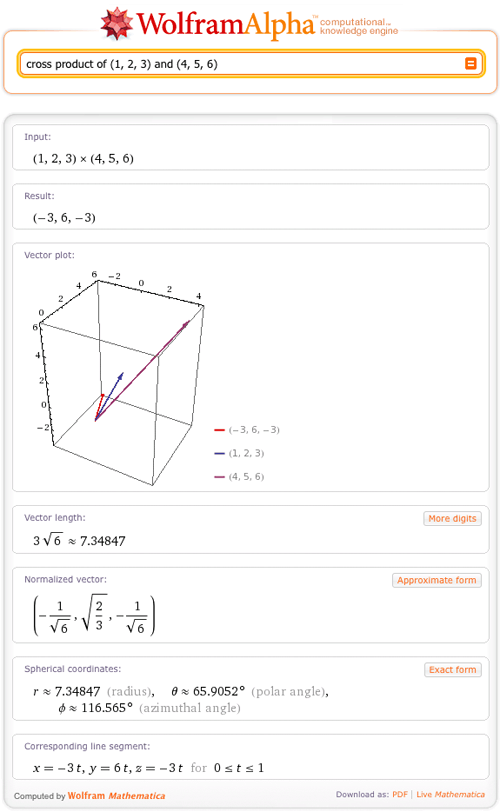TAG: Vectors
June 1, 2011–

What do you get when you cross a mountain climber with a mosquito? Nothing—you can’t cross a scalar with a vector!

But what do you get when you cross two vectors? Wolfram|Alpha can tell you. For example:And in fact, Wolfram|Alpha can give lots of information on vectors. A vector is commonly defined as a quantity with both magnitude and direction and is often represented as an arrow. The direction of the arrow matches the direction of the vector, while the length represents the magnitude of the vector. Wolfram|Alpha can now plot vectors with this arrow representation in 2D and 3D and return many other properties of the vector.
More »